Starting Electronics needs your help! Please make a donation to help cover our hosting and other costs. Click the donate button to send a donation of any amount.# Build a Breadboard Circuit for Beginners

Created on: 12 January 2022

Build a breadboard circuit for beginners in Arduino and electronics. This is a very simple Arduino circuit for a solderless electronic breadboard. Connect the circuit to an Arduino board. After this, control the LED in the circuit by using an Arduino program or sketch. Learn how a solderless electronic breadboard works in this part of the Arduino tutorial for beginners.

Part 6 of the Arduino Tutorial for Beginners

The image below shows the simplest Arduino breadboard circuit. It consists of a resistor, LED and connecting wires.

Complete the previous parts of this Arduino tutorial for beginners before continuing with this part. It is required to have the Arduino IDE software installed and tested on an Arduino board before continuing.

## Build an Easy Arduino Breadboard Circuit

Build an easy Arduino breadboard circuit with a breadboard, LED, resistor and connecting wires or jumpers. The following sub-sections show how to identify electronic components used in the easy breadboard circuit. After this is an explanation on how to build the circuit.

### Identify the Electronic Components

Get the components to be used in the Arduino breadboard circuit together. Firstly get an LED. Secondly get a 470 ohm resistor as described in the following sub-sections. These are components from the first part of this Arduino tutorial for beginners. Find the components in the table of that part of the tutorial.

#### Identify an LED or Light Emitting Diode

LED stands for Light Emitting Diode. In fact, that is a good description of what an LED does. It is a diode that emits light. The image below shows a 5mm blue LED. 5mm is the diameter of the LED bulb. For this example a blue LED is used, but you can change between different colored LEDs during the tutorial.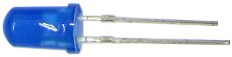Also see the article on LEDs for beginners that has more basic information on Light Emitting Diodes.

#### Identify a 470 Ohm Resistor

Use a 470 ohm resistor in this easy Arduino circuit for beginners. To clarify, a 470 ohm resistor has color bands of yellow, violet and brown. The following image shows a 470 ohm 5% tolerance resistor.Each color band of a resistor has a value. As shown above, yellow has a value of 4, violet is 7 and brown is 1. As can be seen in the image below, the values of resistor color bands are found in the resistor color code chart. The three colored bands on the left of the above resistor represent the value of the resistor in ohms. The first two values represent the first two numbers of the resistance value. In this example these are 4 and 7, so the first part of the resistance value is 47. The third value represents the number of zeros in the resistance value. In this example the value is 1, which means one zero. Place the number of zeros after the first two numbers and we get 470. This means the resistor value is 470 ohms, or 470Ω.

The gold band at the right represents the tolerance of the resistor. That is 5% tolerance for gold.

In addition to the above example, see resistors for beginners in electronics that contains more information on resistors and the resistor color chart. Finally, see the Wikipedia resistor color code for another illustration on how to read resistor values. While the previous example shows a 3 digit 5% tolerance resistor, the Wikipedia resistor color code shows 4 digit 1% tolerance resistors as well.

### Build the Arduino Breadboard Circuit

Bend the legs or pins of the 470 ohm resistor down and plug the resistor into the breadboard as shown in the image below. As can be seen in the image, the resistor straddles the middle channel of the breadboard. This ensures that the resistor leads are not connected together, or shorted out.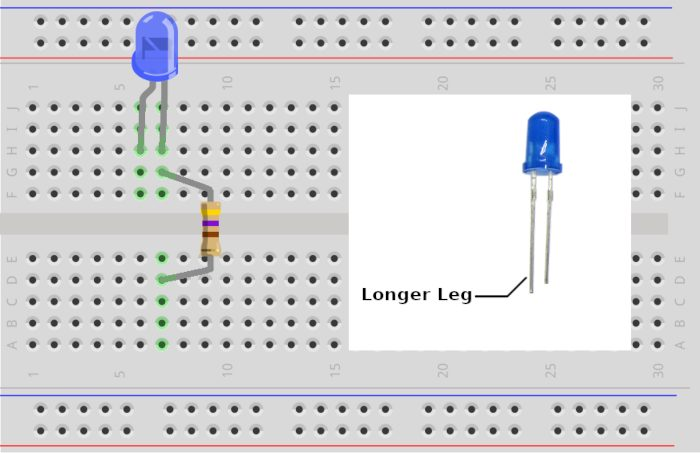Insert the LED into the breadboard as shown in the above image. Most important is inserting the LED the correct way around. One of the LED pins is longer than the other, as the inset in the above image shows. Orient the LED with the longer pin at the left. Of course this results in the short leg of the LED connecting to the resistor.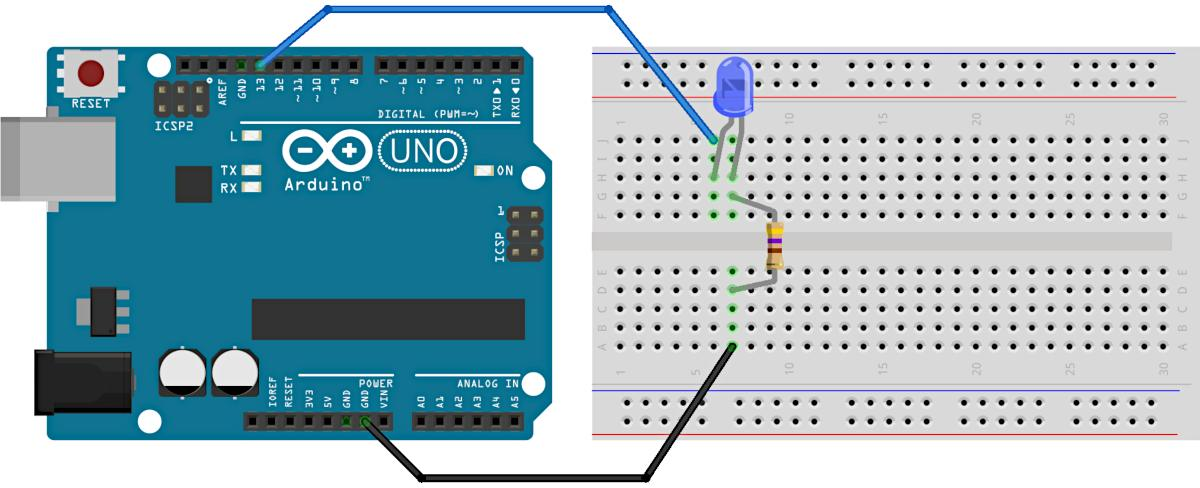Connect the free leg of the resistor to one of the Arduino GND pins. As can be seen above, a black wire connects the free resistor leg to an Arduino Uno GND pin. In the same way, connect the free pin of the resistor to the GND pin of an Arduino MEGA 2560, if using this board. The image below shows the connection to a MEGA 2560.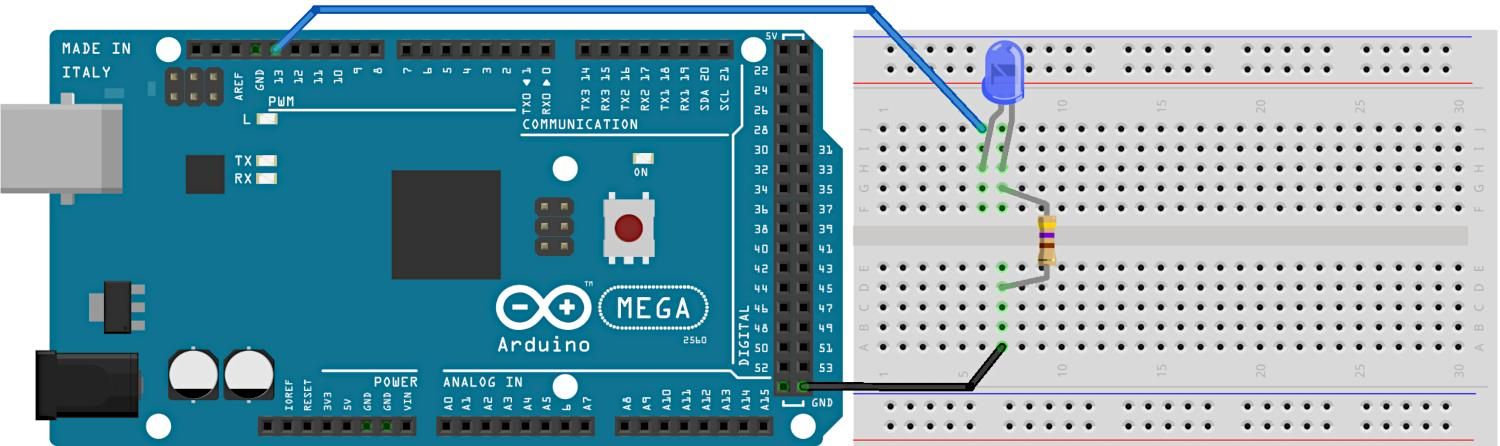Finally connect the long pin of the LED to pin 13 of the Arduino Uno or MEGA 2560. As can be seen in the above two images, a blue wire connects from the free leg of the LED to pin 13 of both Arduino boards. The image below shows a photo of the circuit built using an Arduino Uno compatible board. It does not matter if you use different color wires in your circuit. By convention black is used to represent GND or 0V, but the circuit works if any other color wire is used instead.

### Test the Arduino Breadboard Circuit for Beginners

Double-check that the circuit is built correctly. Plug the Arduino board into the host computer using a USB cable. Load the Arduino Blink example sketch to the target Arduino board. Once again select File → Examples → 01.Basics → Blink from the top Arduino IDE menu to open the Blink sketch. After this, upload the Blink sketch to the target Arduino Board.

The LED on the breadboard blinks on and off along with the on-board LED. That is of course if the circuit is built and connected correctly. In addition it is assumed that the components used are working and not faulty.

If the circuit does not work, then try the following. Firstly check that the on-board LED blinks. Because the on-board LED is connected to pin 13 of the Arduino, it blinks when the Blink sketch runs. As the LED and resistor circuit is also connected to pin 13, it blinks along with the on-board LED. Secondly, check that the wiring of the circuit is correct. Finally check that the LED is oriented the correct way around, as previously explained.

### How a Solderless Electronic Breadboard Works

The image below shows the internal wiring of a solderless electronic breadboard. That is, it shows the connections of the holes or tie-points of the breadboard. To clarify, the green lines in the image represent conductors that conduct electricity.

The image shows that two horizontal connections are found at the top edge of the breadboard. Similarly, two horizontal connections are found at the bottom edge. The middle of the breadboard consists of rows of five holes connected vertically. Vertical connections at the top half of the board are separated from vertical connections at the bottom half of the board. The middle insulating channel separates the two vertical connection halves.

The previous circuit uses only three of the vertical connections. The following image shows the connections made on the breadboard from the previous circuit.

The above image shows the long pin on the left of the LED connected to the blue wire that goes to pin 13 of the Arduino board. Component leads, legs or pins plugged into the holes of the same breadboard conductor are joined together. Another connection is between the right short pin of the LED and the top lead of the resistor. As can be seen in the image, pins from these components are plugged into the holes of the same vertical conductor. The result is that these component pins are electrically connected together. Finally, the bottom lead of the resistor connects to the black wire that goes to the Arduino GND pin.

There are many ways to build the same circuit on a breadboard. For example, the first image at the top of this part of the tutorial shows the same LED resistor circuit built differently. It uses one of the bottom horizontal connections or rails as GND. GND is then connected to the resistor via a green jumper. After this, both the resistor and LED are connected across the lower half of the vertical breadboard connections.

## Circuit Diagrams for Beginners

Circuit diagrams show electrical connections between components of a circuit. Components are represented by symbols in circuit diagrams, rather than images of physical components. For example, the circuit diagram below represents the previously built circuit.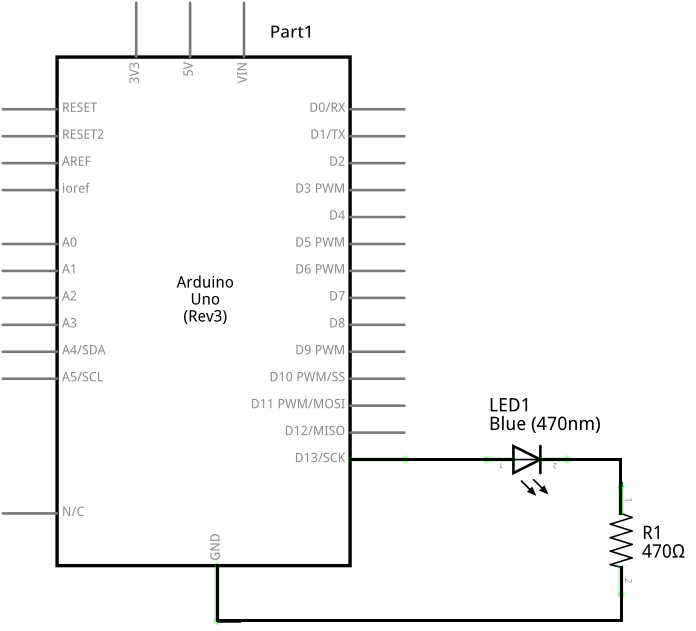As the above image shows, the LED connects to Arduino pin 13. After this, the other pin of the LED connects to the resistor. Finally, the other pin of the resistor connects to the Arduino Uno GND. An LED symbol represents the LED. Likewise, a symbol represents the resistor. A component number, called a reference designator, is placed next to each component. That is, LED1 is the reference designator of the LED, and R1 is the reference designator of the resistor. The Arduino Uno is represented by a complex symbol in the above circuit diagram. The following image shows the same circuit drawn using a simpler symbol for the Arduino Uno. In addition, it uses an alternate symbol for the resistor.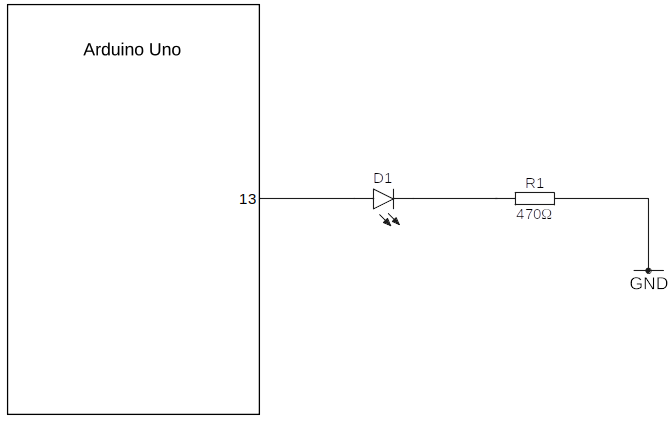The above simpler circuit diagram represents the Arduino as a rectangle with one pin. Because only pin 13 is used in the circuit, only pin 13 is shown in the Arduino Uno symbol. GND connects to the right pin of the resistor. This implies that the resistor connects to a GND pin on the Arduino.

Refer to basic information on LEDs for more on LED symbols and polarity. Also refer to basic information on resistors for different resistor symbols used in circuit diagrams.

In effect, both of the above circuit diagrams mean that the circuit is connected as follows.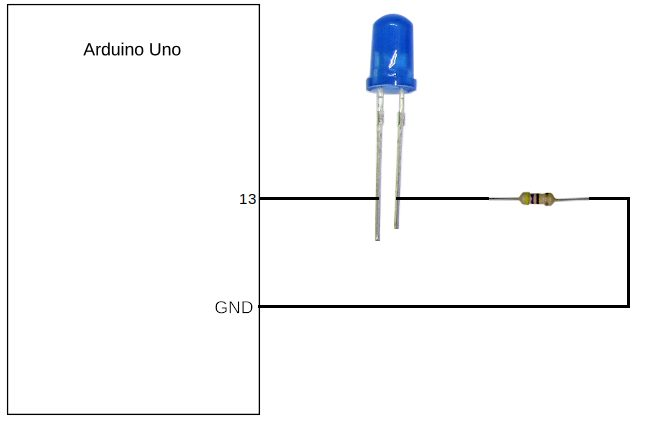## Series LED and Resistor Circuit

In the circuit, the LED and resistor are connected in series. When the Arduino Blink sketch switches pin 13 high, it effectively puts 5V on pin 13. As a result, current flows from pin 13, through the LED, then through the resistor, and finally back to the GND pin. When the Blink sketch switches pin 13 low, it places 0V or GND on the pin. This stops current flowing through the series circuit. As a result, the LED switches off.

Use the same circuit with the PhysicalPixel example sketch from the previous part of this tutorial called Processing Language for Beginners in Arduino. This allows the PhysicalPixel processing application to control the external LED on the breadboard circuit in addition to the Arduino on-board LED.

## Modify the Blink Sketch to use a Different Pin

Modify the Blink sketch to use a different Arduino pin in this last section. Arduino boards have digital pins marked 0 to 13 on an Arduino Uno. Arduino MEGA 2560 boards have these same pins as well as many more. Open the Blink sketch and change the code to use digital pin 2 of the board instead of pin 13, as follows.

Change LED_BUILTIN to 2 in the three places that it is found in the Blink sketch. LED_BUILTIN represents pin 13 on Arduino Uno and Arduino MEGA 2560 boards. Changing it to 2 causes the Blink sketch to set up pin 2 as an output pin. In addition, the Blink sketch now switches pin 2 high and low. Save the sketch as Blink_pin2, or use your own preferred name for the sketch. The following code is the modified blink sketch code without the comments.

``````void setup() {
pinMode(2, OUTPUT);
}

void loop() {
digitalWrite(2, HIGH);
delay(1000);
digitalWrite(2, LOW);
delay(1000);
}``````

Unplug the Arduino board from the USB port. When making changes to a circuit, always disconnect the power first. Use the same LED and resistor series circuit. Instead of connecting the LED to pin 13 of the Arduino Uno or MEGA 2560, connect it to pin 2. Finally, plug the USB cable back into the board and upload the modified sketch. The external LED connected to pin 2 of the Arduino now blinks on and off.

Find much more information on Arduino pins and configuration in the Ultimate Arduino Uno Hardware Manual for Arduino Uno boards. Refer to the Ultimate Arduino MEGA 2560 Hardware Manual for the same information on Arduino MEGA 2560 boards. These books also have information on resistor values to use with LEDs. In addition they cover configurations such as current sourcing and sinking. Not all Arduino pins are available as output pins, especially when used with some shields. The manuals explain which pins to use when.

## Build a Breadboard Circuit for Beginners Conclusion

This part of the Arduino tutorial for beginners covers some of the basics of building Arduino breadboard circuits. In addition, we looked at identifying components and the basics of circuit diagrams. Refer to the article on how to read circuit diagrams for beginners for additional information on reading circuit diagrams.

Building a circuit on breadboard is part of the Start Electronics Now electronics course for beginners. This part of the course shows how to build a simple LED circuit. Follow it using an Arduino to power the circuit. Use the Arduino 5V and GND instead of a 9V battery. Because the circuit is now powered from 5V use a 470 ohm series resistor instead of a 1k resistor.

As an Amazon Associate I earn from qualifying purchases: